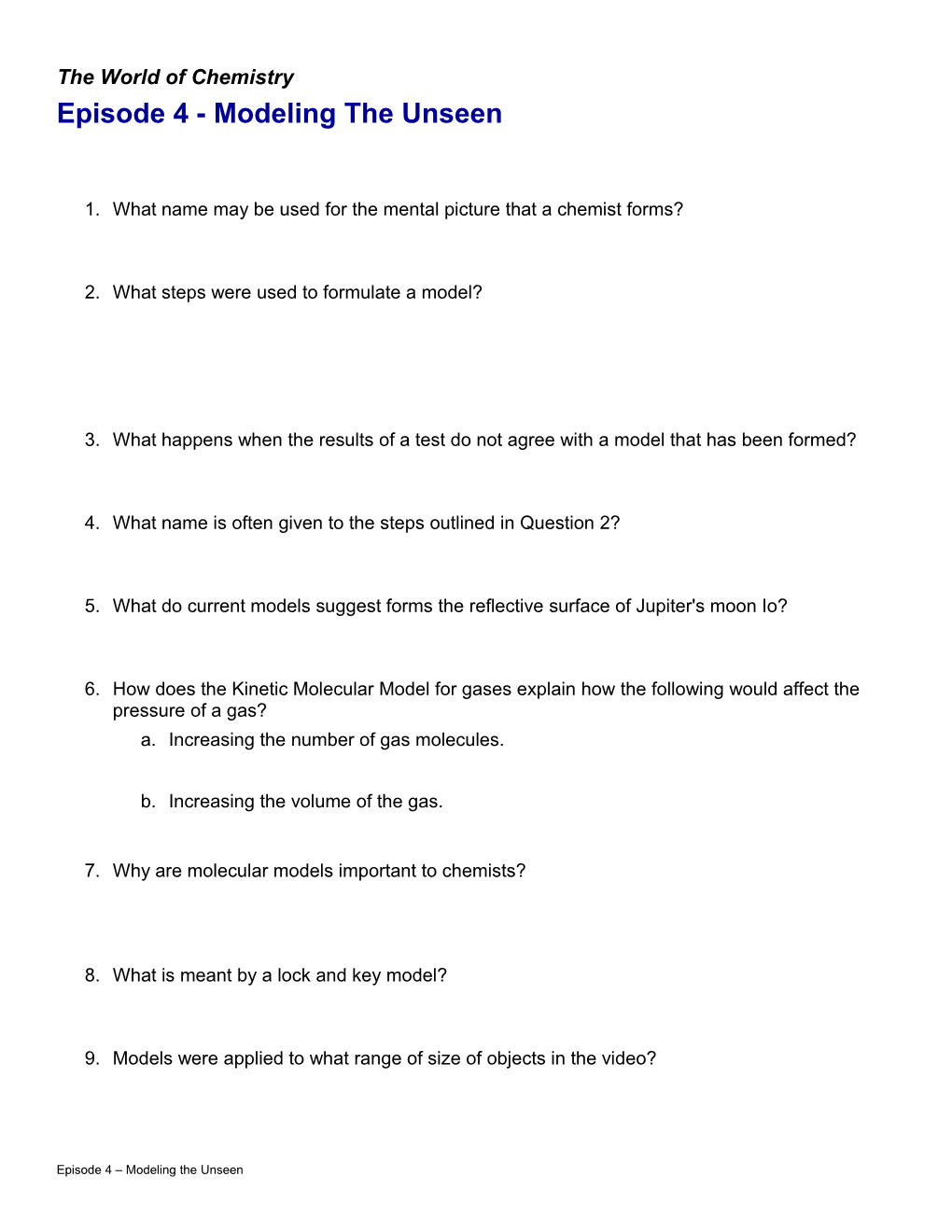# Modeling the UnseenThe World of Chemistry

Episode 4 - Modeling The Unseen

1. What name may be used for the mental picture that a chemist forms?
2. What steps were used to formulate a model?
3. What happens when the results of a test do not agree with a model that has been formed?
4. What name is often given to the steps outlined in Question 2?
5. What do current models suggest forms the reflective surface of Jupiter's moon Io?
6. How does the Kinetic Molecular Model for gases explain how the following would affect the pressure of a gas?
7. Increasing the number of gas molecules.
8. Increasing the volume of the gas.
1. Why are molecular models important to chemists?
2. What is meant by a lock and key model?
3. Models were applied to what range of size of objects in the video?

1. What name may be used for the mental picture that a chemist forms?

Model

1. What steps were used to formulate a model?
2. Make observations
3. Form a model (temporary)
4. Test the model.
5. What happens when the results of a test do not agree with a model that has been formed?

Further testing and observations are made and a new model is formulated.

1. What name is often given to the steps outlined in Question 2?

Scientific method.

1. What do current models suggest forms the reflective surface of Jupiter's moon Io?

It is covered with sulfur or sulfur compounds due to extensive volcanic action.

1. How does the Kinetic Molecular Model for gases explain how the following would affect the pressure of a gas?
2. Increasing the number of gas molecules.

The number of collisions with the sides of the container would increase causing an increase in pressure.

1. Increasing the volume of the gas.

The number of collisions per unit area of the container would decrease resulting in a decrease in pressure.

1. Why are molecular models important to chemists?

They provide a three dimensional model of the molecule which is often more realistic than flat two dimensional drawings. They can be used to make predictions about possible uses for the compound (drugs).

1. What is meant by a lock and key model?

One molecule must fit precisely in another in order for a reaction to occur. This is common in enzymes (catalysts in living systems).

1. Models were applied to what range of size of objects in the video?

They were used from the molecular level to the solar system level (with Io - Jupiter's moon).

Episode 4 – Modeling the Unseen